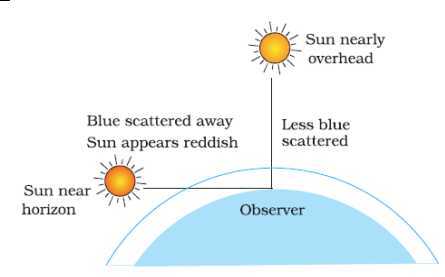# NCERT Solutions

## Class 10 science

###### human eye and colourful world1. The human eye can focus objects at different distances by adjusting the focal length of the eye lens. This is due to

1. presbyopia
2. accommodation
3. near-sightedness
4. far-sightedness

(b)accommodation

2. The human eye forms the image of an object at its

1. cornea
2. iris
3. pupil
4. retina

(d)retina

3. The least distance of distinct vision for a young adult with normal vision is about

1. 25m
2. 2.5cm
3. 25cm
4. 2.5m

(c)25cm

4. The change in focal length of an eye lens is caused by the action of the

1. pupil
2. retina
3. ciliary muscles
4. iris

(c)ciliary muscles

5. A person needs a lens of power -5.5 dioptres for correcting his distant vision. For correcting his near vision he needs a lens of power +1.5 dioptre. What is the focal length of the lens required for correcting (i) distant vision, and (ii) near vision ?

The power P of a lens of focal length f is given by the relationP= 1/f
(i) Power of the lens used for correcting distant vision = - 5.5 D
Focal length of the required lens, f= 1/Pf= 1/-5.5 = -0.181 m
The focal length of the lens for correcting distant vision is - 0.181 m.
(ii) Power of the lens used for correcting near vision = +1.5 D
Focal length of the required lens, f= 1/P
f= 1/1.5 = +0.667 m
The focal length of the lens for correcting near vision is 0.667 m.

6. The far point of a myopic person is 80 cm in front of the eye. What is the nature and power of the lens required to correct the problem?

The person is suffering from an eye defect called myopia. In this defect, the image is formed in front of the retina. Hence, a concave lens is used to correct this defect of vision.
Object distance, u = infinity = ?
Image distance, v = - 80 cm
Focal length = f
According to the lens formula,
Using (1/f) = (1/v) – (1/u)
(1/f) = 1/ (-80) + (1/-infinity)
(1/f) = - (1/80) + (-0)
f = -80 cm
f = -0.8 m
Power = (1/f)
= 1/ (-0.8)
= -1.25 D
The nature of lens required to see the distant objects clearly is convex lens is power -1.25 D.

7.Make a diagram to show how hypermetropia is corrected. The near point of a hypermetropia eye is 1 m.
What is the power of the lens required to correct this defect? Assume that the near point of the normal eye is 25 cm.

It is corrected by using convex lens of suitable focal length.
Near point hypermetropia eye v = -1m = -100cm
Object distance u = -25cm
According to formula, (1/v) – (1/u) = (1/f)
Near point of hypermetropia eye:-
(1/f) = - (1/100) – (1/-25) = (-1/100) + (1/25)
(1/f) = (-1+4)/ (100) = (3/100)
f = (100/3)
Near point of a hypermetropic eye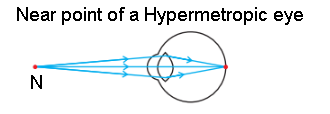Hypermetropia eye:-
f = (100/3)
P = (1/f) = (3 x 100)/ (100) = 3D
A convex lens of power +3.0 D is required to correct the defect.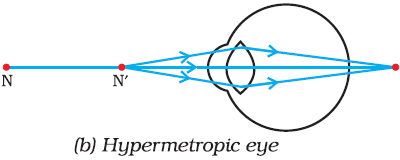Correction of a hypermetropia eye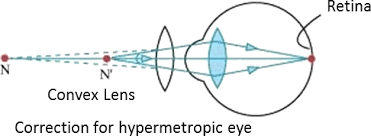8. Why is a normal eye not able to see clearly the objects placed closer than 25 cm?

The image distance in the eye, is fixed and cannot be changed. The ability of the eye lens to adjust its focal length is called accommodation.
Due to this eye can increase or decrease focal length of the lens in order to see either close or distant objects.
In order to see nearby objects the ciliary muscles contract and lens become thick and focal length is decreased which helps to see nearby objects.
In order to see distant objects the ciliary muscles expand and the lens become thin and focal length increases which help to see distant objects.

10.Why do stars twinkle?

Stars twinkle due to atmospheric refraction of starlight. The light from the star on entering earth’s atmosphere undergoes refraction continuously before it reaches the earth.
Refraction occurs and refractive index changes.
As the path of rays of light coming from the star goes on varying slightly, the apparent position of the star fluctuates and the amount of starlight entering the eye flickers.
Therefore sometimes star appears brighter and sometimes twinkle.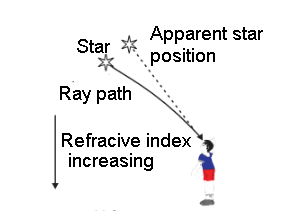11.Explain why the planets do not twinkle.

Planets, do not twinkle as they are closer to the Earth than those distant stars, so planets appear larger in comparison. Due to the planets’ closeness to Earth, the light coming from them does not bend much due to Earth’s atmosphere. Therefore, the light coming from our solar system’s planets does not appear to twinkle.

12.Why does the Sun appear reddish early in the morning?